# Protein Binding Models

#### 2021-03-28

When collecting protein-ligand binding data using a technique such as Biolayer Interferometry (BLI) or Surface Plasmon Resonance (SPR), it is useful to simulate binding curves to help optimise your experiments. After initial binding parameters are known, binding curves can be simulated and parameters such as: analyte concentration, time of association, dissociation etc. can be varied. The models within this package may also be used to fit a curve to measured binding data using a non-linear regression.

Currently, two binding models are included with this package:

• 1:1 binding
• 2:1 heterogeneous binding.

## 1:1 Binding

Usage: binding1to1(t, t0, conc, kon, koff, rmax)

$Response = \frac{[A]R_{max}}{[A] + K_{D}}(1 - e^{-(K_{on}[A] + K_{off})t})$

Parameters:

• $$t$$, time (s) - can also be a vector.
• $$t_0$$, time of dissociation.
• $$conc$$, concentration of analyte in M.
• $$k_{on}$$ $$(k_a)$$, units $$M^{-1}s^{-1}$$
• $$k_{off}$$ $$(k_d)$$, units $$s^{-1}$$
• $$R_{max}$$, maxiumum response.
library(pbm)
time <- seq(0, 1000)
response <- binding1to1(time, 500, 6e-7, 10000, 0.01, 0.8)
plot(time, response, type = "l")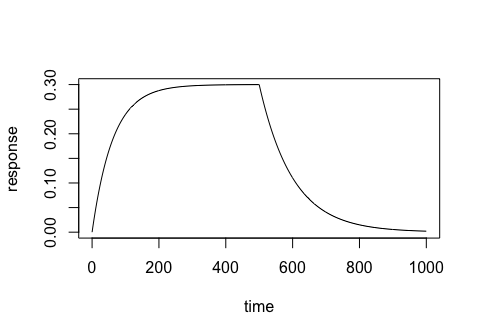Example of a 1:1 binding curve.

### Optional drift parameter

library(pbm)
time <- seq(0, 1000)
response <- binding1to1(time, 500, 6e-7, 10000, 0.01, 0.8, drift = 1e-04)
#> Warning in binding1to1(time, 500, 6e-07, 10000, 0.01, 0.8, drift = 1e-04): Drift
#> parameter set
plot(time, response, type = "l")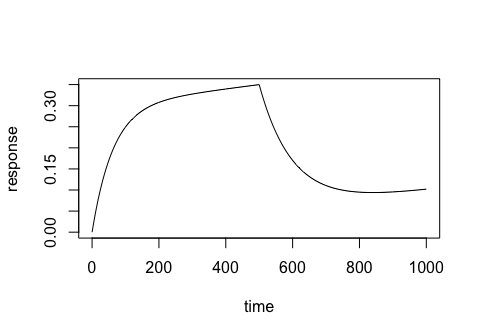Example of a 1:1 binding curve with baseline drift.

## 2:1 Binding

library(pbm)
time <- seq(0, 1000)
response <- binding2to1(time, 500, 6e-7, 10000, 0.01, 0.5, 2500, 0.001, 0.3)
plot(time, response, type = "l")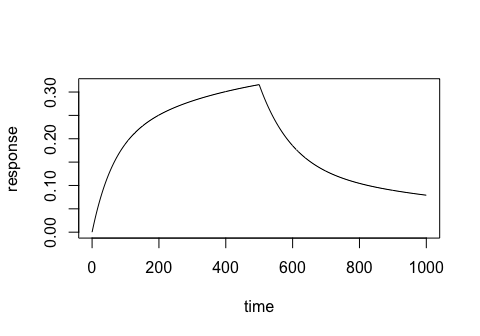Example of a 2:1 binding curve.

## Non-linear Regression

library(pbm)

# Generate example binding data with noise
time <- seq(0, 1000)
response <- binding2to1(time, 500, 6e-7, 10000, 0.01, 0.5, 2500, 0.001, 0.3)
noisyresponse <- jitter(response, amount = 0.02)
data <- data.frame(time, noisyresponse)
names(data) <- c("x", "y")

# Fit a nlm to binding data
startingvalues <- list(kon1 = 70000, koff1 = 0.01, rmax1 = 0.3, kon2 = 9000, koff2 = 0.004, rmax2 = 0.3)
fit <- nls(y ~ binding2to1(x, 500, 6e-7, kon1, koff1, rmax1, kon2, koff2, rmax2),
data = data,
start = startingvalues)

# Plot the fitted model
plot(data$x, data$y, type = "p", pch = 4, cex = 0.5)
par(col = "red", lwd = 3)
lines(data$x, predict(fit, list(x = data$x)))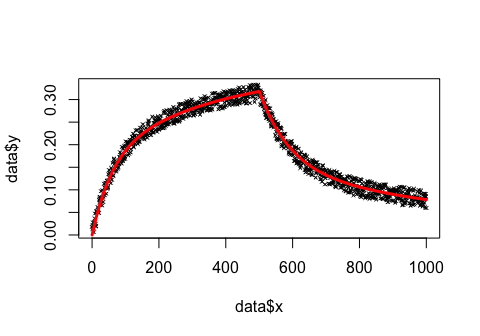Example of 2:1 heterogeneous model fit.

Parameters predicted from fitted model:

kon1 koff1 rmax1 kon2 koff2 rmax2
10163.93 0.0108452 0.4813816 2262.95 0.0012512 0.3817

## Estimating ideal association times

When collecting data, it is recommended to allow the associtaion to reach equilibrium. The command tteq(), by default, returns the time taken to reach 95% equilibrium. See below for an example usage.

# Choose a range of analyte concentrations and give known parameters.
conc_range <- c(6e-7, 3e-7, 1.75e-7, 8.75e-8, 2.916e-8)
kon  <- 10000
koff <- 0.01

# Calculate the time to equilibrium for each concentration.
tteq(conc_range, kon, koff)
#>  187.2333 230.4409 254.9559 275.4696 291.0852

Now we can see that our time to equilibrium for the given concentrations ranges from 187 seconds to 291 seconds. Given these values we can see the difference using calculated data. Below are curves for the given parameters with two different association times.

library(ggplot2)
library(gridExtra)

t <- seq(0, 300)
t0 <-  median(t)
plot1 <- ggplot()
for (conc in conc_range) {
curve <- binding1to1(t, t0, conc, 10000, 0.01, 1)
plot1 <- plot1 + geom_line(aes_string(x = t, y = curve))
}

t <- seq(0, 600)
t0 <-  median(t)
plot2 <- ggplot()
for (conc in conc_range) {
curve <- binding1to1(t, t0, conc, 10000, 0.01, 1)
plot2 <- plot2 + geom_line(aes_string(x = t, y = curve))
}

plot1 <- plot1+labs(x = "Time (s), t0 = 150")
plot1 <- plot1+labs(y = "Response")
plot2 <- plot2+labs(x = "Time (s), t0 = 300")
plot2 <- plot2+labs(y = "Response")

grid.arrange(plot1, plot2, ncol = 2)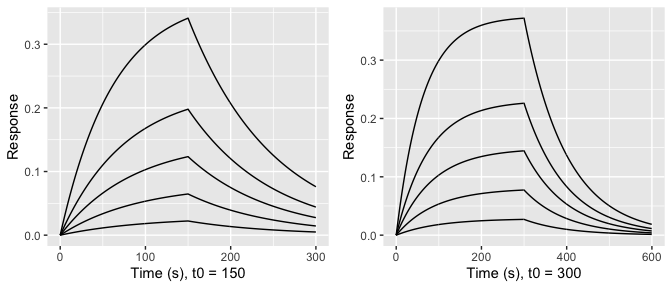Selecting an appropriate association time.